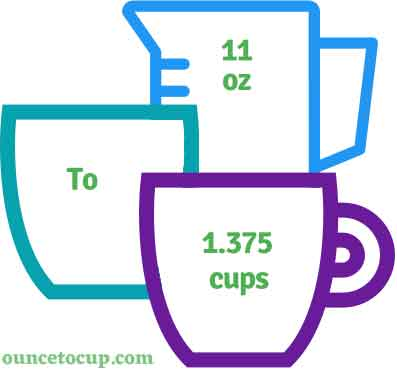# 11 Ounce to Cups (11 oz to cups conversion)

Are you cooking your favorite dish? The detailed chart in the recipe includes the calculation of 11 ounces to cups conversion? Do not worry; check this conversion tool to find how many 11 ounces equal to cups in a minute. This 11 oz to cup converter gives an exact measurement for any recipe you prepare.

Ounce Value:

Ounces

Cup Value:

Cups

11 Ounce = 1.375 Cup
(11 oz = 1.375 cup)

Try our auto 11 ounce to cup calculator (Without Convert Button), Just change the first field value and you got final value.## How many cups is 11 oz?

We know that the volume value of 11 oz is equal to 1.375 cups. If you want to convert 11 fluid oz to an equal number of cups, just divide the volume value by 8. Hence, 11 Ounce is equal to 1.375 cup.

The Answer is: 11 US Fluid Ounces = 1.375 US Cup

11 oz = 1.375 cup

Many of them try to search or find an answer for what is 11 ounces in cups? So, we’ll start with 11 fl oz to cups conversion to know how big is 11 oz.

## How To Calculate 11 fluid oz to cups?

To calculate 11 fluid ounces to an equal number of cups, simply follow the steps below.

Fluid Ounces to Cups formula is:

Cup = Fluid Ounce / 8

Assume that we are finding out how many cups were found in 11 fl oz of water, multiply it either by 0.125 or divide the value by 8 to get the result.

Applying to Formula: Cup = 11 oz / 8 = 1.375 cup.

## How to convert 11 oz to cups?

• To convert 11 fluid ounces to cups,
• Simply multiply the 11 fluid ounces by 0.125, or simply divide them by 8.
• Applying to the formula, cups = 11 ounces * 0.125 [11x0.125] (or) cups = 11 ounces/8 = 11/8 = 1.375.
• Hence, 11 ounces is equal to 1.375 cups.

## Some quick table references for fluid ounce to cup conversions:

Ounce [oz] Cup [c]
1 oz0.125 cup
2 oz0.25 cup
3 oz0.375 cup
4 oz0.5 cup
5 oz0.625 cup
6 oz0.75 cup
7 oz0.875 cup
8 oz1 cup
9 oz1.125 cup
10 oz1.25 cup
11 oz1.375 cup
12 oz1.5 cup

## Reverse Calculation: How many ounces are in 11 cups?

• To convert 11 cups to ounces,
• Simply multiply the 11 cups by 8.
• Then, applying the formula, ounce = 11 cup * 8 [11x8 = 88].
• Hence, 11 cup is equal to 88 ounces.